# How to Find and Replace Values Greater Than or Less Than A Fixed Value in Excel

Suppose we have a list of scores, and we want to replace values equal and greater than 60 to text ‘PASS’, this work cannot be implemented by ‘Find and Replace’ function due to this function is only used for replacing a fixed value to another fixed value. Actually, we can edit VBA code to implement this special ‘Find and Replace’. Setting a fixed value in code, then after running macro, all values greater than this fixed value can be replaced with another value properly. This article will show you the details.

Precondition:

See screenshot below. We want to replace values >=60 with text ‘PASS’.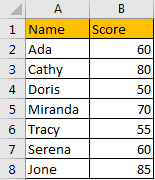## Method: Find and Replace Values Greater Than or Less Than A Fixed Value by VBA Code

Step 1: On current visible worksheet, right click on sheet name tab to load Sheet management menu. Select View Code, Microsoft Visual Basic for Applications window pops up.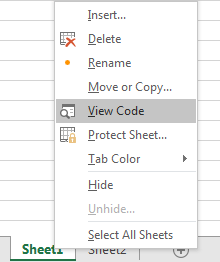Or you can enter Microsoft Visual Basic for Applications window via Developer->Visual Basic. You can also press Alt + F11 keys simultaneously to open it.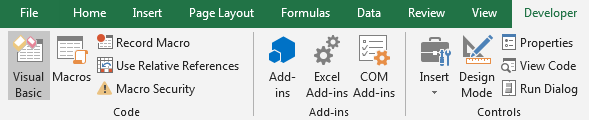Step 2: In Microsoft Visual Basic for Applications window, click Insert->Module, enter below code in Module1:

```Sub FindAndReplace()

Dim UpdateRng As Range

Dim SelectRng As Range

Set SelectRng = Application.Selection

Set SelectRng = Application.InputBox("Select  a Range", "Find and Replace", SelectRng.Address, Type:=8)

For Each UpdateRng In SelectRng

If UpdateRng.Value >= 60 Then

UpdateRng.Value = "PASS"

End If

Next

End Sub```

Step 3: Save the macro and then quit Microsoft Visual Basic for Applications.

Step 4: Click Developer->Macros to run Macro.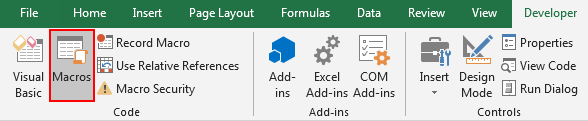You can also press Alt+F8 to trigger Macro directly.

Step 5: Select ‘FindAndReplace’ and click Run.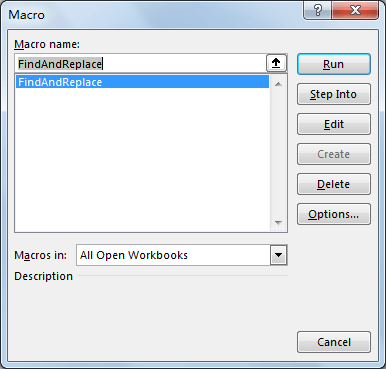Step 6: FindAndReplace dialog pops up. Enter Select Range \$B\$2:\$B\$8. In this step you can select the range you want to replace values.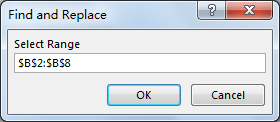Step 7: Click OK. Verify that values equal to or greater than 60 are replaced with ‘PASS’ properly.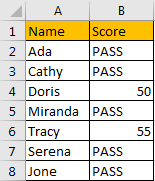Comment:

If you want to find and replace values less than a fixed value, just change below code:

```For Each UpdateRng In SelectRng

If UpdateRng.Value >= 60 Then

UpdateRng.Value = "PASS"

End If

Next

To:

For Each UpdateRng In SelectRng

If UpdateRng.Value < 60 Then

UpdateRng.Value = "FAIL"

End If

Next```

Related Posts

If Cell is This Value or That Value

IF function is frequently used in Excel worksheet to return you expect “true value” or “false value” based on the result of logical test. If you want to see if a cell is A or B, and if one of ...

If Value is Greater Than A Certain Value

IF function is frequently used in Excel worksheet to return you expect “true value” or “false value” based on the logical test result. If you want to see if a value in one cell is greater than a specific value, ...

If Cell is Not Blank

IF function is frequently used in Excel worksheet to return you expect “true value” or “false value” based on the result of created logical test. If you want to see if a cell is blank or not, and leave some ...

VBA Macro For VLOOKUP From Another Sheet

In the previous post, you should know that how to fix or remove the #N/A error when using VLOOKUP formula to lookup value from another sheet. And this post will show you how to use VBA code to vlookup data ...

If Cell is Blank

IF function is frequently used in Excel worksheet to return you expect “true value” or “false value” based on the result of created logical test. If you want to see if a cell is blank or not, and leave some ...

If Cell Equals Certain Text String

IF function is frequently used in Excel worksheet to return you expect “true value” or “false value” based on the result of created logical test. If you want to see if cell equals a certain text string like “Win”, you ...

If Cell Contains Either Text1 or Text2

IF function is frequently used in Excel worksheet to return “true value” or “false value” based on the logical test result. If you want to see if cell contains certain substring1 like “abc” or substring2 like “def”, and returns true ...

If Cell Contains Certain Text OR Equals Certain Text

IF cell equals certain text IF function is frequently used in Excel worksheet to return “true value” or “false value” based on the logical test result. If you want to test values to see if they equal certain text like ...

VLOOKUP From Another Sheet Not Working

In the previous post, you should know that how to fix or remove the #N/A error when using VLOOKUP formula to lookup value from another sheet. And this post will show you reasons why your VLOOKUP formula is not working ...

If Cell Begins with One of Three Supplied Characters

If you want to test values to see if they begin with some given specific characters like “x”, ”y”, or “z”, you can create a formula with COUNTIF and SUM functions to return results. EXAMPLE You can see “TRUE” or ...

Sidebar